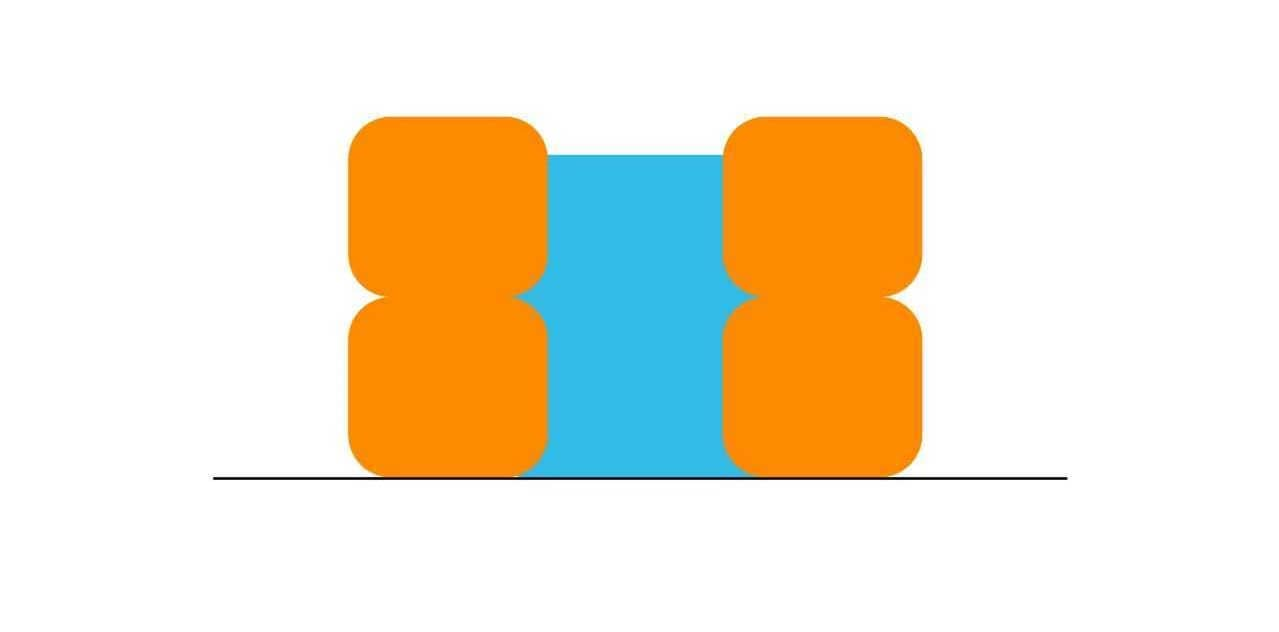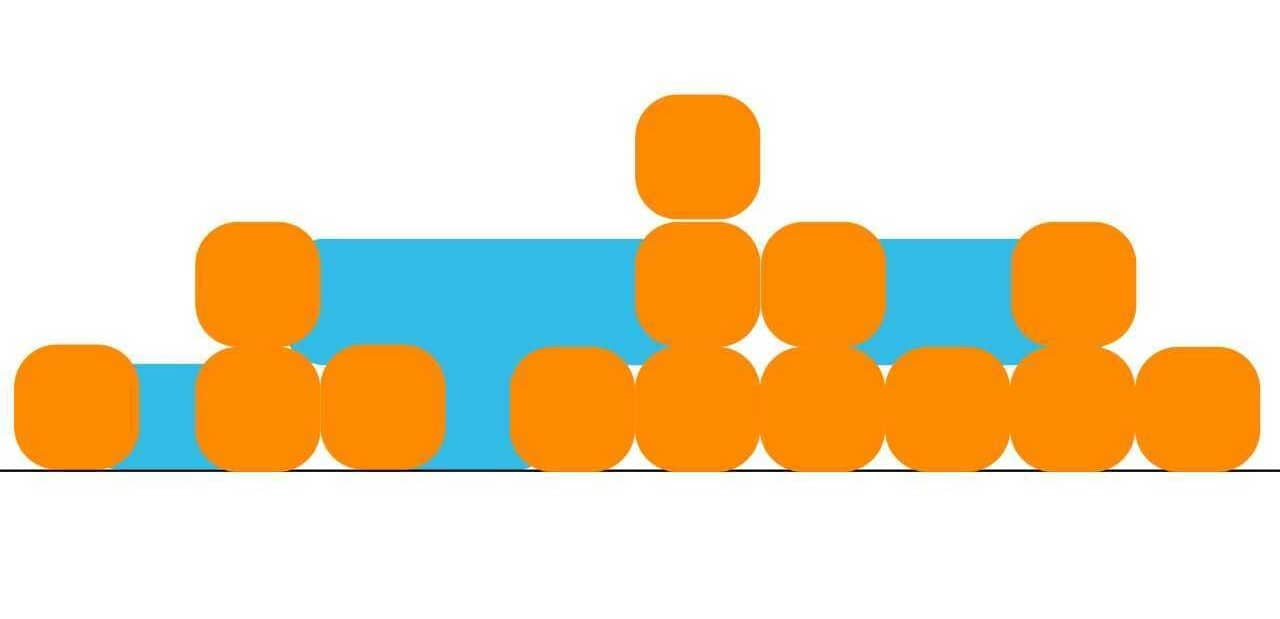# Solving Trapping Rain Water Problem (with C++ & Java code)

As the number of applicants is increasing year after year, the companies don't have any other option than to increase the standards of their hiring patterns. After analyzing past trends, one thing is sure instead of asking direct questions on algorithms and data structures, companies have started asking questions based on real-life experiences and famous puzzles.

With this being said, in today's tech blog, we present to you yet another situational problem that is based almost on real life. Now, as the topic suggests we are going to understand the Trapping Rain Water Problem which was recently asked about by tech giants like Meta and Oracle.

We'll go through the problem, understand what the interviewer is actually asking from us with help of examples, know the intuition behind solving the problem, and finally the code implementation of various methodologies of the solution.

So, what are you waiting for? You're in for a fantastic ride!

## Trapping Rain Water Problem Statement

Here is the problem statement: "You are given an array of non-negative integers arr[]. It represents an elevated map where the width of each bar is 1. You have to calculate the amount of water that can be trapped inside it."

Let's look at an example.

### Example

Input: arr[]={2, 0, 2}

Output: 2

Explanation: You can take a look at the given illustration to understand the given example.Input: arr[]={0, 1, 0, 2, 1, 0, 1, 3, 2, 1, 2, 1}

Output: 6

Explanation: Take a look at the given illustration to understand the given example.### Understanding the Problem

After looking at the above examples, we are sure that you must have understood what the problem actually wants you to do. If you are still facing any issues, let's understand the problem. So, in this section, we'll look through what the problem is.

So, in this problem, you're given an array that has only positive values. Now, you need to visualize the array elements as horizontal blocks and later, imagine how much water can be fitted into the gaps of these blocks. Now, after this, you'll have to add the value in between and return it as the answer.

TIP: Use paper and pen to visualize it initially.

## Trapping Rain Water Problem Solution

In this section, we'll understand how to actually solve this problem with various techniques.

### Method 01) Naive Approach

Follow the given algorithm to implement the given method:

```1. Traverse the array from beginning to end:
2. For every element:
-Traverse the array from start to that index and find the maximum height (h1)
-Traverse the array from the present index to the end, and find the maximum height (h2).
3. The volume of water that will be stored in this column is min(h1,h2) – array[i], add the value to total water stored.
```

### Method 02) Precalculation

Follow the given algorithm to implement the given method:

```1. Create two arrays left[] and right[] of size n.
2. Create a variable (say maximum) to store the maximum water till a certain index during traversing the array.
3. Run one loop from beginning to end:
-In each iteration update maximum and also assign left[i] = maximum.
4. Run another loop from end to beginning:
-In each iteration update maximum found till now and also assign right[i] = maximum.
5. Traverse the array from beginning to end.
6. The total amount of water that will be stored in this column is min(left[i], right[i]) – array[i]
7. The value that came will be added to the total water amount.
```

### Method 03) Stack

Follow the given algorithm to implement the given method:

```1. Loop through the indices of the bar array.
2. For each bar, do the following steps:
-Loop while the Stack is not empty and the current bar has a height greater than the top bar of the stack,
-Store the index of the top bar in pop_height and pop it from the Stack.
-Find the distance between the left bar(current top) of the popped bar and the current bar.
-Find the minimum height between the top bar and the current bar.
-The maximum water that can be trapped is distance * min_height.
-The water trapped, including the popped bar, is (distance * min_height) – height[pop_height].
3. Return the amount received as the total amount of water
```

### Method 04) Horizontal Scan Method

Follow the given algorithm to implement the given method:

```1. Find the total number of blocks, i.e., the sum of the heights array, num_blocks
2. Find the maximum height, max_height
3. Store total water in a variable, total = 0
4. Keep two pointers, left = 0 and right = N -1, to store the leftmost and the rightmost boundaries for each unit of height
5. For each height, i from 1 to max_height, do the following
6. Find the leftmost and the rightmost boundary for the current height.
7. As mentioned earlier we can consider all the blocks in between these to have at least i unit of water.
8. Add this amount of water to the total trapped water.
9. After the iteration is over, subtract num_blocks from total as we have considered them as water height during calculation.
```

### Method 05) 2-pointer Method

Follow the given algorithm to implement the given method:

```1. Take two pointers l and r.
2. Initialize l to the starting index 0 and r to the last index N-1.
3. Since l is the first element, left_max would be 0, and right_max for r would be 0.
While l ≤ r, iterate the array.
4. We have two possible conditions
Condition1 : left_max <= right max
-Consider Element at index l
-Since we have traversed all elements to the left of l, left_max is known
-For the right max of l, We can say that the right max would  always be >= current r_max here
-So, min(left_max,right_max) would always equal to left_max in this case
-Increment l.

Condition2 : left_max > right max
-Consider Element at index r
-Since we have traversed all elements to the right of r, right_max is known
-For the left max of l, We can say that the left max would  always be >= current l_max here
-So, min(left_max,right_max) would always equal to right_max in this case
-Decrement r.
```

### Method 06) Using Linear Search to some extent

Follow the given algorithm to implement the given method:

```1. Loop from index 0 to the end of the given array.
-If a bar greater than or equal to the previous bar is encountered, then store the index of that bar (say prev_index).
-Keep adding the previous bar’s height minus the current (ith) bar to the final answer.
-Have a temporary variable to store the amount of water in the current segment.
-If no bar greater than or equal to the previous bar is found, then quit.
2. If prev_index < size of the input array, then subtract the temporary variable from the answer, because we have considered the last segment that has no higher right bound.
-Loop from the end of the input array to prev_index.
-Find a bar greater than or equal to the previous bar (in this case, the last bar from backward).
```

## Complexity Analysis

 S.No. Method Time Complexity Space Complexity 1. Naive Approach O(n²) O(1) 2. Precalculation O(n) O(n) 3. Stack O(n) O(n) 4. Horizontal Stack O(max (max_height, n)) O(1) 5. 2-pointer O(n) O(1) 6. Linear Search O(n) O(1)

where, n=size of array

## Code Implementation with C++

Here is the C++ code to solve the Trapping Rain Water Problem:

```#include <bits/stdc++.h>
using namespace std;

int maximumWater(int arr[], int n)
{
int size = n - 1;
int prev = arr;

int prev_index = 0;
int wat = 0;
int temp = 0;
for (int i = 1; i <= size; i++) {

if (arr[i] >= prev) {
prev = arr[i];
prev_index = i;
temp = 0;
}
else {
wat += prev - arr[i];
temp += prev - arr[i];
}
}

if (prev_index < size) {

wat -= temp;
prev = arr[size];
for (int i = size; i >= prev_index; i--) {
if (arr[i] >= prev) {
prev = arr[i];
}
else {
wat += prev - arr[i];
}
}
}
return wat;
}
int main()
{
int arr[] = { 0, 1, 0, 2, 1, 0, 1, 3, 2, 1, 2, 1 };
int n = sizeof(arr) / sizeof(arr);
cout << maximumWater(arr, n);
return 0;
}
```

## Code Implementation with Java

Here is the Java code to solve the Trapping Rain Water Problem:

```class Solution {
public static int maximumWater(int arr[], int n)
{
int size = n - 1;
int prev = arr;
int prev_index = 0;
int water = 0;
int temp = 0;
for (int i = 1; i <= size; i++) {
if (arr[i] >= prev) {
prev = arr[i];
prev_index = i;
temp = 0;
}
else {
water += prev - arr[i];
temp += prev - arr[i];
}
}
if (prev_index < size) {
water -= temp;
prev = arr[size];
for (int i = size; i >= prev_index; i--) {
if (arr[i] >= prev) {
prev = arr[i];
}
else {
water += prev - arr[i];
}
}
}
return water;
}
public static void main(String[] args)
{
int arr[] = { 0, 1, 0, 2, 1, 0, 1, 3, 2, 1, 2, 1 };
int n = arr.length;
System.out.print(maximumWater(arr, n));
}
}```

```6
```

## Conclusion

So, we have discussed a total of 6 methods for you to solve this problem. All the methods are equally valid but, in terms of complexity, you can take a look at our complexity analysis and select a suitable method yourself. We are sure that you must have understood this problem and the next time, if it comes to your interview you'll pass with flying colors.

### FavTutor - 24x7 Live Coding Help from Expert Tutors!